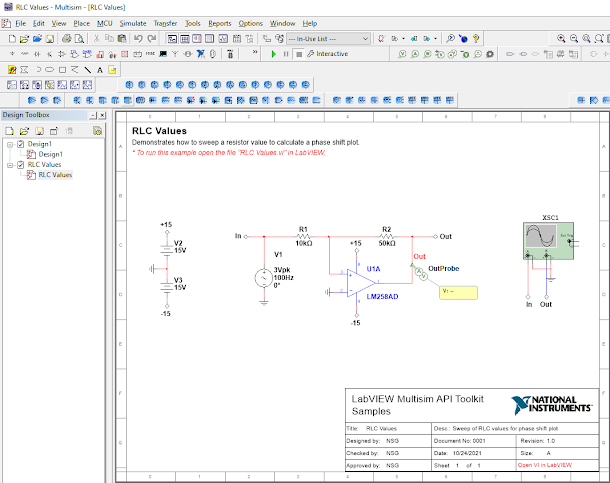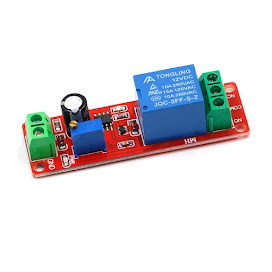Showing posts with label simulation. Show all posts
Showing posts with label simulation. Show all posts

## Sunday, October 24, 2021

### LabVIEW Multisim API Toolkit - RLC Values Example

Written by: Larsha Johnson
Date: October 24th, 2021

If you like designing electronic circuits in Multisim and would like to automate the simulation and analyses in LabVIEW, this example will walk you through how it can be done. The link below may help with missing NI module, Toolkit, or driver warning when opening VI errors.

Multisim Toolkit with LabView

The Multisim Automation API and the LabVIEW Multisim API Toolkit (which uses the API) recognize and can be used to control the values of all circuit parameters in the design. This lets you control most component parameters from the API.

This example uses Multisim version 14.2 and LabVIEW 2021

First open Multisim file RLC values from the blue sample folder → LabView Multisim API Toolkit.After the file is loaded into Multisim workspace, open LabVIEW, click the "File" tab and open the RLC Values vi as shown.

The simulation should run from here if all the necessary NI Modules, toolkits, and drivers are installed correctly. However, we will explore further by clicking the "Windows" tab and opening the associated block diagram. *The original design was modified by removing the blocks section "Multisim preparation" and replaced with a single "Select File Path" block form the Multisim Add-on tab.

💬This may differ depending on software version.

If any errors should occur, in this diagram by block location is where they will be listed. From the LabVIEW front panel or from the block diagram, press the white arrow icon to run the simulation on the Multisim RLC Values circuit.

This demonstrates how to sweep a resistor value to calculate a phase shift plot. (set to R2 by default)

In Multisim alternative analyses and simulations can be performed such as "Parameter Sweep", "Transfer Function", and "Pole Zero" to name a few.

🤔 Enjoyed this blog? Learned something useful? Consider following us for more content.

If you have errors visit this link: NI LABS TOOLKITS or comment if you find solutions to help others.

https://www.ieee.li/pdf/viewgraphs/circuit_design_simulation_and_virtual_instrumentation.pdf

## Saturday, September 25, 2021

### MATLAB - Family of Curves (Vectorization)

Linear Signals and System, matrix operation fundamentals. This example illustrates a techniques called vectorization. Algorithm vectorization uses matrix and vector operations to avoid manual repetition and loop structures. With simultaneous creation of curves one can maximize the use of matrix applications.
The result here is a 201 x 11 matrix with identical columns.

%% Matrix Operations - Family of Curves
r = [1 0 0]
A = [2 3;4 5;0 6]
c = r' B = [c A]
B(1,2)
B(1:2,2:3)
B(2,:)
A = [1 -2 3;-sqrt(3) 1 -sqrt(5);3 -sqrt(7) 1];
y = [1;pi;exp(1)];
x = inv(A)*y x1 = det([y,A(:,2:3)])/det(A) alpha = (0:10);
t = (0:0.001:0.2)';
T = t*ones(1,11);
H = exp(-T*diag(alpha)).*sin(2*pi*10*T+pi/6);
plot(t,H); xlabel('t'); ylabel('h(t)');
[R,P,K] = residue(B,A)
[R,P,K] = residue([1 0 0 0 0 pi],[1 -sqrt(8) 0 sqrt(32) -4]);
R.', P.', K title('Family of Curves - Vectorization')

Try this code out to graph this figure! Modify freely and comment on this post if it helps. Thanks for reading.

### 3 Handy Electronic Component Modules for Your Workbench

There are tons of electronic modules available. Here are three of our favorites available in our Tindie Marketplace store now. These three m...